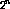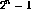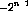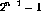Next: Boolean Algebra Up: Number Systems Previous: BinaryOctal and Hexadecimal

## Number Representation

We define the following

word: a binary number consisting of an arbitrary number of bits.
nibble: a 4-bit word (one hexadecimal digit).
byte: an 8-bit word.

We often use the expressions 16-bit word (short word) or 32-bit word (long word) depending on the type of computer being used. Most fast computers today actually employ a 64-bit word at the hardware level.

If a word has n bits it can representdifferent numbers in the range 0 to. Negative numbers are usually represented by the so called 2's complement notation. To obtain the 2's compliment of a number first take the complement (invert each bit) and then add 1. All the negative numbers will have a 1 in the MSB position, and the numbers will now range fromto. The electronic advantages of the 2's complement notation becomes evident when addition is performed. Convince yourself of this advantage.

Doug Gingrich
Tue Jul 13 16:55:15 EDT 1999